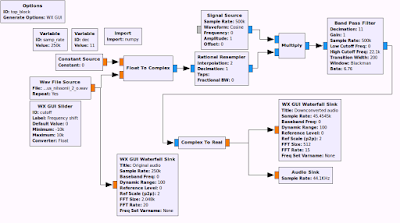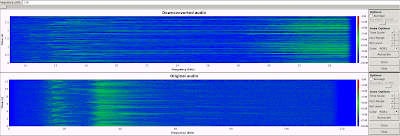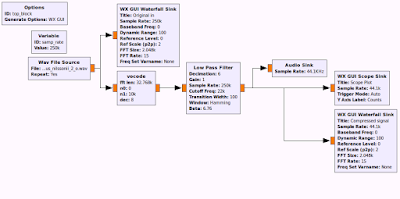### Audible playback of ultrasound using GNURadio Companion

Inspired by the preamp and hydrophone post yesterday by Mikko Syrjäsuo, I put together some gnuradio scripts for processing infra or ultrasound recordings. There are three different flowgraphs: The first one allows you to playback a recording with a faster or slower speed. The second one allows you to shift signal in frequency by multiplying it with a complex sinusoid up or down in frequency to an audible band. The third one is something similar to what Mikko was describing. It uses a custom python block to compress a wide bandwidth into a more narrow bandwidth by using something called a vocoder (at least that is what I think it is called). I've attached the code.

The audio downconversion script and the vocoder script can also be operated in realtime, by replacing the wav-source block with an audio card block. It sounds very weird talking to a microphone and hearing your own voice shifted to a higher or lower frequency.

For more serious applications, you could also use the USRP N2x0 or USRP1 together with the LFRX daughterboard to record ultrasound signals.

I managed to find nice high frequency (250 kHz) ultrasound recordings of bat sounds on this web site: http://www.batcalls.com/. I have used these for testing. Specifically this wav file.Flowgraph to adjust the speed of the audio recording, resulting in sped up or slowed down playback of the wav file.Audio frequency shifting using mixing and filtering. This allows "mixing" the ultrasound onto an audible frequency band, retaining the temporal characteristics of the signal.
Here is an audio clip of the frequency shifted bat call.View of the downconversion gnuradio program in action when listening to a bat recording. Above: audible 0..22.05 kHz waterwall spectrum of the downconverted audio, Below: the 0..125 kHz ultrasound recording.The vocoder flowgraph. The vocode block is a custom block that I wrote in Python. The code is shown below.Bat call signal between 10 and 75 kHz is converted into a 1 to 14 kHz signal.
Here is an audio clip of the vocoder output.
The code for the vocoder is quite simple. It does windowed FFTs, averages frequency bins and shifts them onto audible frequency bands. The overlapping tapered windows are needed in order for the output signal to be continuous. You can find the gnuradio flowgraphs (under examples) and thevocoder block on github.

The code for the vocoder is shown below:
``` ```
``````
``````
``#  `Simple vocoder gnuradio block, (c) 2017 Juha Vierinen `
`#`
``````import numpy as np

class vocode(gr.sync_block):
"""
docstring for block vocode
"""
def __init__(self, n,n0,n1,dec):
self.buflen=4*n+8192
self.tmp_in = np.zeros(self.buflen,dtype=np.float32)  # buffering
self.tmp_out = np.zeros(self.buflen,dtype=np.float32) ```# buffering       ```
``````        self.fin = np.zeros(n,dtype=np.float32)
self.n = n                               # fft size
self.n0 = n0                             # first bin (mapped to dc freq)
self.n1 = n1                             # last bin (mapped to dc+(n1-n0) freq)
self.w=np.zeros(n,dtype=np.float32)      # window function
l2=int(n/2)
self.w[0:l2]=np.linspace(0,1.0,num=l2)
self.w[l2:n]=np.linspace(1.0,0.0,num=l2)``````
``````        # how much do we integrate and decimate spectral components toghether
self.dec = dec            ``````
``````        # some buffer indices
self.idx_bufin  = 0 # location on input buffer
self.idx_proc   = 0 # location on processing buffer
self.idx_out = 0    # location on output buffer

gr.sync_block.__init__(self,
name="vocode",
in_sig=[np.float32],
out_sig=[np.float32])

def work(self, input_items, output_items):
in0 = input_items
out = output_items

L=len(in0)
``````
``````        # insert data into input buffer
self.tmp_in[np.mod(self.idx_bufin+np.arange(L),self.buflen)]=in0
``````
``````
``````
``        # number of windows we can process``
``````        tail = self.idx_bufin+L
n_win = int(np.floor((tail-self.n-self.idx_proc)/(self.n/2)))``````
``````        # process all available FFT windows (half overlapping)
for i in range(n_win):
# window
i0=self.idx_proc
fin=self.tmp_in[np.mod(i0+np.arange(self.n),self.buflen)]
# compress and shift spectrum
F=np.fft.rfft(self.w*fin)
Fc=np.convolve(F,np.repeat(1.0/float(self.dec),self.dec),mode="same")
idxs=np.arange(self.n0,self.n1,self.dec)
Fc2=np.copy(F) ; Fc2[:]=0.0
Fc2[np.arange(len(idxs))]=Fc[idxs]
self.tmp_out[np.mod(i0+2*self.n+np.arange(self.n),self.buflen)]+=self.w*np.fft.irfft(Fc2)

# empty tail of buffer
self.tmp_out[np.mod(i0-self.n+np.arange(self.n),self.buflen)]=0.0
self.idx_proc+=self.n/2``````
``````
self.idx_bufin+=L
out[:]=self.tmp_out[np.mod(self.idx_out+np.arange(L),self.buflen)]
self.idx_out+=L
return L

``````
``` ```